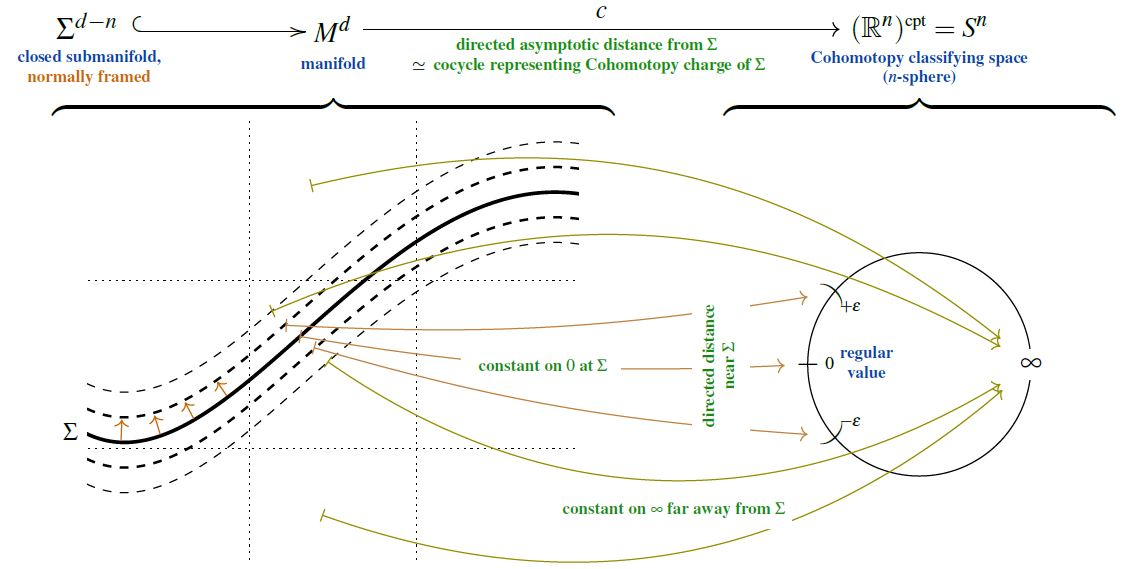# nLab Pontryagin's theorem

Contents

### Context

#### Differential geometry

synthetic differential geometry

Introductions

from point-set topology to differentiable manifolds

Differentials

V-manifolds

smooth space

Tangency

The magic algebraic facts

Theorems

Axiomatics

cohesion

tangent cohesion

differential cohesion

graded differential cohesion

singular cohesion

$\array{ && id &\dashv& id \\ && \vee && \vee \\ &\stackrel{fermionic}{}& \rightrightarrows &\dashv& \rightsquigarrow & \stackrel{bosonic}{} \\ && \bot && \bot \\ &\stackrel{bosonic}{} & \rightsquigarrow &\dashv& \mathrm{R}\!\!\mathrm{h} & \stackrel{rheonomic}{} \\ && \vee && \vee \\ &\stackrel{reduced}{} & \Re &\dashv& \Im & \stackrel{infinitesimal}{} \\ && \bot && \bot \\ &\stackrel{infinitesimal}{}& \Im &\dashv& \& & \stackrel{\text{étale}}{} \\ && \vee && \vee \\ &\stackrel{cohesive}{}& ʃ &\dashv& \flat & \stackrel{discrete}{} \\ && \bot && \bot \\ &\stackrel{discrete}{}& \flat &\dashv& \sharp & \stackrel{continuous}{} \\ && \vee && \vee \\ && \emptyset &\dashv& \ast }$

Models

Lie theory, ∞-Lie theory

differential equations, variational calculus

Chern-Weil theory, ∞-Chern-Weil theory

Cartan geometry (super, higher)

# Contents

## Idea

The Pontryagin theorem (Pontryagin 38a, 50, 55 II.6) identifies, for a closed smooth manifold $M^d$

with

• the $n$-Cohomotopy of $M^d$ – the homotopy classes of maps from $M^d$ into the n-sphere;

viaFrom SS 2021

In this form, with the assumption that $M^d$ is closed, hence compact, the statement appears for instance in Kosinski 93, Sec. IX Prop. 5.5.

More generally, if the smooth manifold $M^d$ is not assumed to be compact, essentially the same Pontryagin-Thom construction still gives an identification of the cobordism classes of its normally framed submanifolds with the reduced Cohomotopy of its one-point compactification:

This form of Pontryagin’s theorem seems to be folklore (e.g. here). It is made fully explicit in Csépai 20, p. 12-13.

An analogous statement, identifying cobordism classes of normally oriented submanifolds with homotopy classes of maps into the universal special orthogonal Thom space $M SO(n)$, is Thom's theorem (Thom 54):

(Now the notion of asymptotic directed distance depends on the normal tangent spaces, along $\Sigma$, which themselves vary now in the Grassmannian $Gr_n$, hence in the classifying space $B SO(n) \subset M SO(n)$.)

Both statements, Pontryagin’s and Thom’s, as well as their joint generalization to other tangential structures (besides framing and orientation structure) and notably their stabilization to Whitehead-generalized Cobordism cohomology theory, have all come to be widely known as the Pontryagin-Thom construction, or similar, a term commonly used also for rather more involved cases, such as in MUFr-theory. This type of construction constitutes the basis of modern cobordism theory and its application in stable homotopy theory.

### Pontrjagin-Thom construction

#### Pontrjagin’s construction

##### General

The Pontryagin theorem, i.e. the unstable and framed version of the Pontrjagin-Thom construction, identifying cobordism classes of normally framed submanifolds with their Cohomotopy charge in unstable Borsuk-Spanier Cohomotopy sets, is due to:

(both available in English translation in Gamkrelidze 86),

as presented more comprehensively in:

The Pontrjagin theorem must have been known to Pontrjagin at least by 1936, when he announced the computation of the second stem of homotopy groups of spheres:

• Lev Pontrjagin, Sur les transformations des sphères en sphères (pdf) in: Comptes Rendus du Congrès International des Mathématiques – Oslo 1936 (pdf)

Review:

Discussion of the early history:

##### Twisted/equivariant generalizations

The (fairly straightforward) generalization of the Pontrjagin theorem to the twisted Pontrjagin theorem, identifying twisted Cohomotopy with cobordism classes of normally twisted-framed submanifolds, is made explicit in:

A general equivariant Pontrjagin theorem – relating equivariant Cohomotopy to normal equivariant framed submanifolds – remains elusive, but on free G-manifolds it is again straightforward (and reduces to the twisted Pontrjagin theorem on the quotient space), made explicit in:

• James Cruickshank, Thm. 5.0.6, Cor. 6.0.13 in: Twisted Cobordism and its Relationship to Equivariant Homotopy Theory, 1999 (pdf, pdf)
##### In negative codimension

In negative codimension, the Cohomotopy charge map from the Pontrjagin theorem gives the May-Segal theorem, now identifying Cohomotopy cocycle spaces with configuration spaces of points:

• Peter May, The geometry of iterated loop spaces, Springer 1972 (pdf)

• Graeme Segal, Configuration-spaces and iterated loop-spaces, Invent. Math. 21 (1973), 213–221. MR 0331377 (pdf)

c Generalization of these constructions and results is due to

• Dusa McDuff, Configuration spaces of positive and negative particles, Topology Volume 14, Issue 1, March 1975, Pages 91-107 (doi:10.1016/0040-9383(75)90038-5)

• Carl-Friedrich Bödigheimer, Stable splittings of mapping spaces, Algebraic topology. Springer 1987. 174-187 (pdf, pdf)

#### Thom’s construction

Thom's theorem i.e. the unstable and oriented version of the Pontrjagin-Thom construction, identifying cobordism classes of normally oriented submanifolds with homotopy classes of maps to the universal special orthogonal Thom space $M SO(n)$, is due to:

Textbook accounts:

#### Lashof’s construction

The joint generalization of Pontryagin 38a, 55 (framing structure) and Thom 54 (orientation structure) to any family of tangential structures (“(B,f)-structure”) is first made explicit in

and the general statement that has come to be known as Pontryagin-Thom isomorphism (identifying the stable cobordism classes of normally (B,f)-structure submanifolds with homotopy classes of maps to the Thom spectrum Mf) is Lashof 63, Theorem C.

Textbook accounts:

Lecture notes:

• John Francis, Topology of manifolds course notes (2010) (web), Lecture 3: Thom’s theorem (pdf), Lecture 4 Transversality (notes by I. Bobkova) (pdf)

• Cary Malkiewich, Section 3 of: Unoriented cobordism and $M O$, 2011 (pdf)

• Tom Weston, Part I of An introduction to cobordism theory (pdf)

See also:

### Cohomotopy in topological data analysis

The suggestion to regard cobordism theory of iso-hypersurfaces and thus Pontryagin's theorem in Cohomotopy as a tool in (persistent) topological data analysis (improving on homologuical well groups):